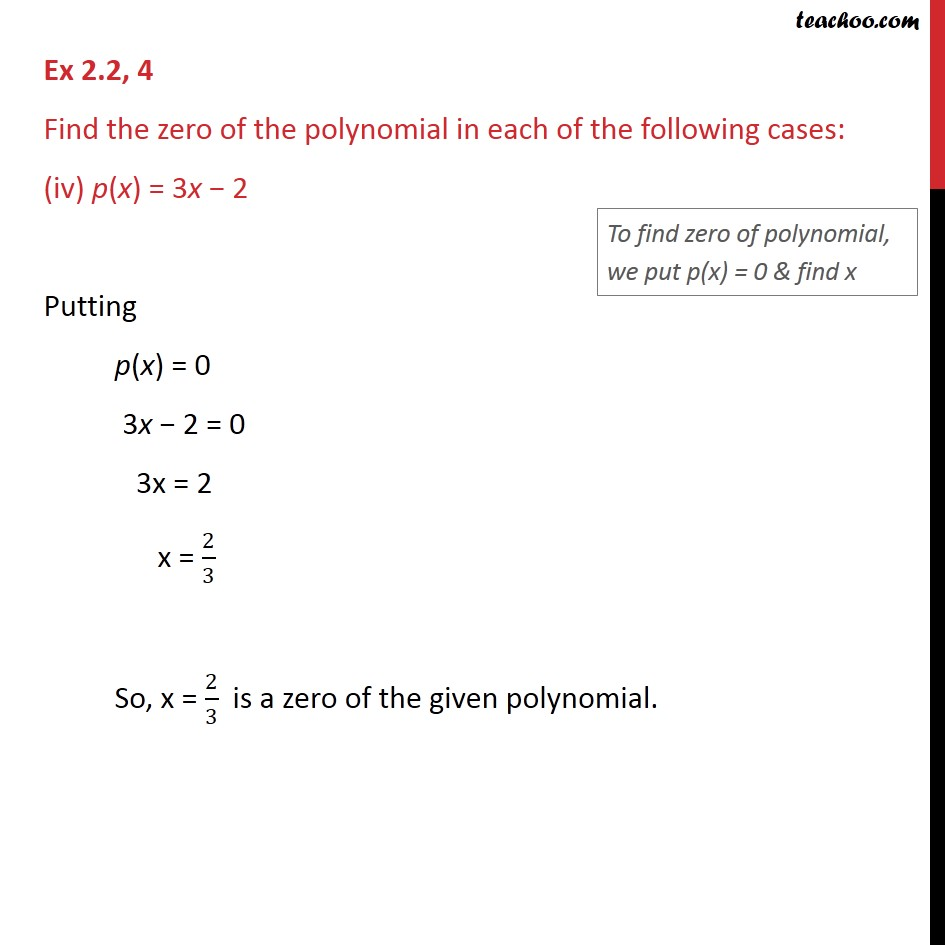Ex 2.2

Chapter 2 Class 9 Polynomials
Serial order wiseLearn in your speed, with individual attention - Teachoo Maths 1-on-1 Class

### Transcript

Ex 2.2, 4 Find the zero of the polynomial in each of the following cases: (iv) p(x) = 3x − 2 Putting p(x) = 0 3x − 2 = 0 3x = 2 x = 2/3 So, x = 2/3 is a zero of the given polynomial.